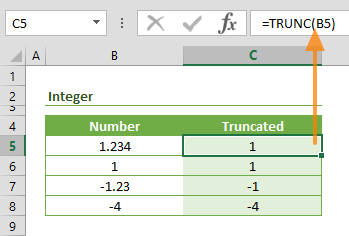The Excel TRUNC function is a Math formula that truncates a given number by removing a number of digits. Although, it is similar to the INT function, the TRUNC function works differently when truncating negative numbers. In this guide, we’re going to show you how to use the TRUNC function and also go over some tips and error handling methods.

## Supported versions

• All Excel versions

## Excel TRUNC function Syntax

TRUNC(number, [num_digits])

## Arguments

 number The number you want to truncate. [num_digits] Optional. The number of fractional digits to be left after truncation. The default value is 0.

## Examples

### Integer Values

The most common use case of the TRUNC function is for getting the integer part of a number. If [num_digits] is omitted, the function truncates the number into an integer. For example,

=TRUNC(1.234) returns 1

=TRUNC(-1.234) returns -1### Decimal Values

Alternatively, the TRUNC function can be used to return a number with its decimal places without rounding it. The [num_digits] argument defines the number of decimal digits you want to extract.

For example,

=TRUNC(1.567,2) returns 1.56

The function excludes any digits after the [num_digits].## Summary and Tips

• While the TRUNC function truncates the number, the INT function rounds the number down to get its integer part. This difference doesn't make a difference when working with positive numbers. However, the integer part will be different when a negative number is rounded down.
=INT(-1.567) returns -2
• To return fractional numbers by rounding, use rounding functions like ROUND, ROUNDUP, or ROUNDDOWN.